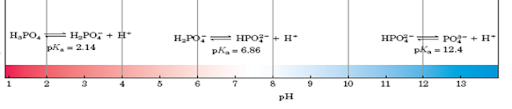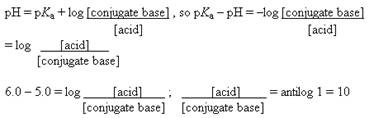# pH Practice Problems with Answers

1. Phosphoric acid (H3PO4) has three dissociable protons, with the pKa’s shown below. Which form of phosphoric acid predominates in a solution at pH 4?
Acid    pKa
H3PO4 2.14
H2PO4 6.86
HPO42– 12.4Ans: At pH 4, the first dissociable proton (pKa = 2.14) has been titrated completely, and the second (pKa = 6.86) has just started to be titrated. The dominant form at pH 4 is therefore H2PO4, the form with one dissociated proton.

2. What is the pH of a solution containing 0.2 M acetic acid (pKa = 4.7) and 0.1 M sodium acetate?
Ans:
pH = pKa + log (conjugate base/ acid)
pH = 4.7 + log (0.1/0.2)
= 4.7 – 0.3
pH = 4.4

3. For a weak acid with a pKa of 6.0, show how you would calculate the ratio of acid to salt at pH 5.
Ans:4. Suppose you have just added 100 mL of a solution containing 0.5 mol of acetic acid per liter to 400 mL of 0.5 M NaOH. What is the final pH?
(The pKa of acetic acid is 4.7.)

Ans: Addition of 200 mmol of NaOH (400 mL ´X 0.5 M) to 50 mmol of acetic acid (100 mL X 0.5 mM) completely titrates the acid so that it can no longer act as a buffer and leaves 150 mmol of NaOH dissolved in 500 mL, an [OH] of 0.3 M.
Given [OH], [H+] can be calculated from the water constant:
[H+][OH] = 10–14
[H+] = 10–14 M2 / 0.3 M
pH is, by definition, log (1/[H+])
pH = log (0.3 M /10–14 M2) = 12.48.

5. A weak acid HA, has a pKa of 5.0. If 1.0 mol of this acid and 0.1 mol of NaOH were dissolved in one liter of water, what would the final pH be?
Ans: Combining 1 mol of weak acid with 0.1 mol of NaOH yields 0.9 mol of weak acid and 0.1 mol of salt.
pH = 5.0 + log (0.1/0.9)
pH = 4.05

1.2.